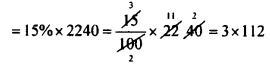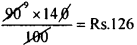# KSEEB Solutions for Class 8 Maths Chapter 9 Commercial Arithmetic Ex 9.3

Students can Download Maths Chapter 9 Commercial Arithmetic Ex 9.3 Questions and Answers, Notes Pdf, KSEEB Solutions for Class 8 Maths helps you to revise the complete Karnataka State Board Syllabus and score more marks in your examinations.

## Karnataka Board Class 8 Maths Chapter 9 Commercial Arithmetic Ex 9.3

Question 1.
An article marked Rs. 800 is sold for Rs. 704. Find the discount and discount percent.
M.P = Rs. 800.
S.P = Rs. 704
Discount = MP – S.P = 800 – 704 = Rs. 96
Discount percentage =$\frac { Discount }{ M.P }$ × 100$\frac { 90 }{ 800 }$ × 100 = 12%

Question 2.
A dress is sold at Rs. 550 after allowing a discount of 12%. Find its marked price.
S.P. = Rs. 550
Rate of discount = 12%
Let the marked price be Rs. 100.
Then S.P = 100 – 12 = 88
If S.P. isRs. 88 then the marked price is Rs.100
If the S.P. is Rs. 550 the M.P. is$\frac{100 \times 550}{88}=\frac{55000}{88}$ = 625
Marked price = Rs. 625.

Question 3.
A shop keeper buys a suit piece for Rs. 1400 and marks it 60% above the cost price. He allows a discount of 15% on it. Find the marked price of the suit piece and also the discount is given.
C.P = Rs. 1400.
M.P = 60% above the C.P.
M.P = 1400 + 60% of 1400M.P= Rs. 2240
Discount = Rate of discount × M.P.Discount = Rs. 336.

Question 4.
A dealer marks his goods 40% above the cost price and allows a discount of 10%. Find the profit percent.
Let the cost price be Rs. 100.
Then marked price is 100 + 40 = Rs. 140.
Rate of discount = 10%
If the marked price is Rs.100 the S.P. is 100 – 10 = Rs. 90
If the marked price is Rs. 140 the S.P.
=∴ C.P. = Rs. 100
S.P. = Rs. 126
S.P > C.P
∴ There is profit Profit = 126 – 100 = Rs. 26
Profit percentage = 26% [∴ C.P. is 100]

Question 5.
A dealer is selling an article at a dis¬count of 15%. Find
(i) The selling price if the market price is Rs. 500
(ii) The cost price if he marks a 25% profit.
$$\frac { 85 }{ 100 }$$ × 500